• 一篇关于麦克风阵列声源定位的论文，具有一定的参考价值。
•研究论文
•研究论文
• ## 麦克风阵列声源定位 GCC-PHAT

万次阅读 多人点赞 2018-03-28 22:16:43
麦克风阵列声源定位 利用麦克风阵列可以实现声源到达方向估计（direction-of-arrival (DOA) estimation），DOA估计的其中一种方法是计算到达不同阵元间的时间差，这里主要介绍经典的GCC-PHAT方法 背景 简单说明...
麦克风阵列声源定位（一）
利用麦克风阵列可以实现声源到达方向估计（direction-of-arrival (DOA) estimation），DOA估计的其中一种方法是计算到达不同阵元间的时间差，另外一种可以看这里，这篇主要介绍经典的GCC-PHAT方法
背景
简单说明问题背景，信号模型如下图，远场平面波，二元阵列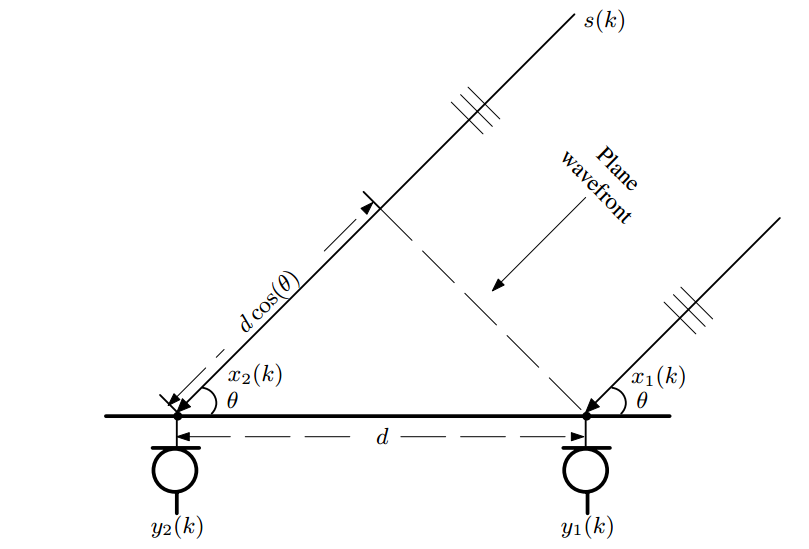要计算得到θ\theta，其实就是要求两个阵元接收到的信号时间差，现在问题变成到达时间差估计（Time-Difference-of-Arrival Estimation），因此，基于延时估计的DOA方法，其实也可以看做是分两步进行的，第一步是估计延时，第二步是计算角度，与之相对应的基于空间谱估计的DOA方法就是一步完成的。下面就分两步进行介绍
##1.延时估计
###1.1.互相关函数（cross-correlation function
计算y1(k)y_1(k)与y2(k)y_2(k)的时间差，可以计算两个信号的互相关函数,找到使互相关函数最大的值即是这两个信号的时间差
离散信号的互相关函数
R(τ)=E[x1(m)x2(m+τ)]R(\tau)=E[x_1(m)x_2(m+\tau)]
求时间差就是找到互相关函数最大时的点
D=argmaxR(n)D=argmaxR(n)
说的那么简单，那就用代码验证下
%%
c = 340.0;
Fs = 44100;
%%

d = 0.25;
N = 2;
mic = phased.OmnidirectionalMicrophoneElement;
% array = phased.URA([N,N],[0.0724,0.0418],'Element',mic);
array = phased.ULA(N,d,'Element',mic);

%%
% Simulate the incoming signal using the |WidebandCollector| System
% object(TM).
arrivalAng = 42;
collector = phased.WidebandCollector('Sensor',array,'PropagationSpeed',c,...
'SampleRate',Fs,'ModulatedInput',false);
signal = collector(y,arrivalAng);

x1 = signal(:,1);
x2 = signal(:,2);

N =length(x2);
xc = xcorr(x1,x2,'biased');
[k,ind] = max(xc);
an = acos((ind-N)/Fs*340/d)*180/pi

xc12 = zeros(2*N-1,1);
m = 0;
for i = -(N-1):N-1
m = m+1;
for t = 1:N
if 0<(i+t)&&(i+t)<=N
xc12(m) = xc12(m) + x2(t)*x1(t+i);
end
end
end
xc12 = xc12/N;

以上程序中的循环就是上面的定义公式，运行程序可以看到循环部分计算的互相关与直接调用matlab的xcorr结果相同（注意matlab中互相关默认没做归一化）,找到互相关函数的最大值就可以得到时间差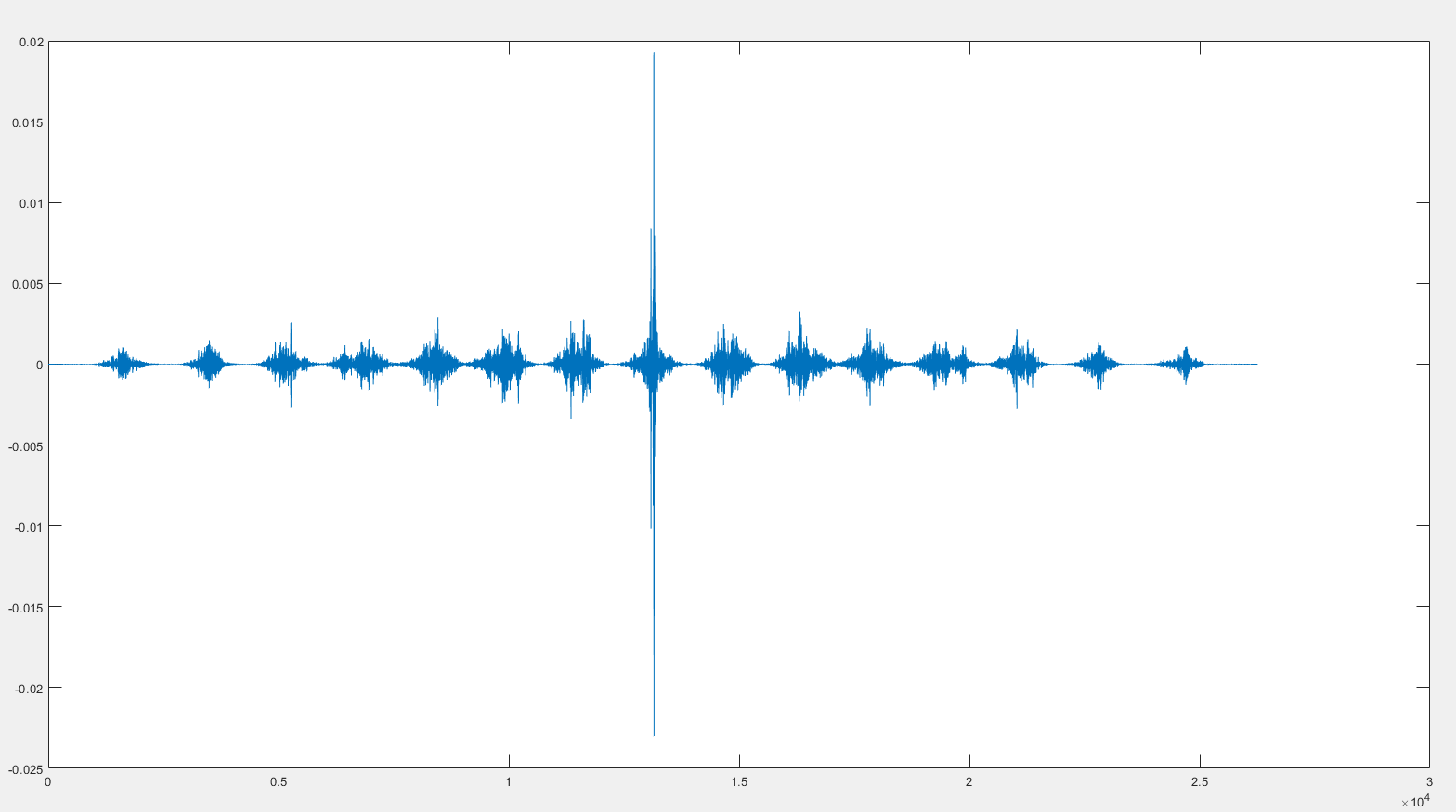1.2.广义互相关（generalized cross-correlation）
理论上使用上面个介绍的CCF方法就可以得到时间差，但是实际的信号会有噪声，低信噪比会导致互相关函数的峰值不够明显，这会在找极值的时候造成误差。
为了得到具有更陡峭极值的互相关函数，一般在频域使用一个加权函数来白化输入信号，这就是经典的广义互相关方法。
由维纳-辛钦定理可知，随机信号的自相关函数和功率谱密度函数服从一对傅里叶变换的关系，即x1、x2x_1、x_2的互功率谱可由下式计算
P(ω)=∫−∞+∞R(τ)e−jωτdτP(\omega)=\int_{-\infty }^{+\infty }R(\tau)e^{-j\omega\tau}d\tau
R(τ)=∫−∞+∞P(ω)ejωτdωR(\tau)=\int_{-\infty }^{+\infty }P(\omega)e^{j\omega\tau}d\omega
这一步是把互相关函数变换到了频域，哦对，上面说到是想白化互相关函数，那就把上面第二式添加一个系数
R~(τ)=∫−∞+∞A(ω)P(ω)ejωτdω\tilde{R}(\tau)=\int_{-\infty }^{+\infty }A(\omega)P(\omega)e^{j\omega\tau}d\omega
设计不同的频域系数A(ω)A(\omega)对应着不同方法，这里只介绍 PHAT(phase transform)方法，即取系数如下：
A(ω)=1∣P(ω)∣A(\omega) = \frac{1}{\left | P(\omega) \right |}
基本思想就是求时间差只需要相位信息，舍弃不相关的幅度信息以提高健壮性，可以看到当A(ω)=1A(\omega)=1的情况下就是经典互相关P(ω)P(\omega)为复数，可以表示为∣P(ω)∣∗e−jωp\left |P(\omega)\right |*e^{-j\omega p},去掉幅度信息后，就只剩相位信息e−jωpe^{-j\omega p}了，要得到相位信息，可以用 P(ω)abs(P(ω))\frac{P(\omega)}{abs(P(\omega))}计算，也可以直接用matlab中的angle函数计算，即angle(P(ω))angle(P(\omega))，
具体得到更陡峭的峰值的理论解释如下,详情参见《麦克风阵列信号处理》P198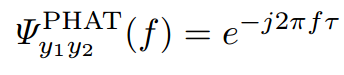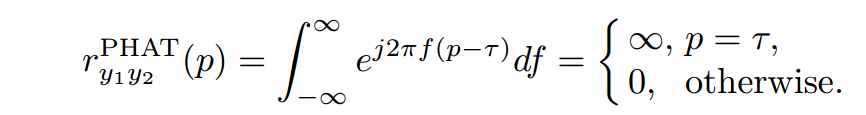几行代码验证下：
x1 = [1,2,3,7,9,8,3,7]';
x2 = [4,5,6,5,4,3,8,2]';

[tau,R,lag] = gccphat(x1,x2)

N = length(x1)+length(x2)-1;
NFFT = 32;
P = (fft(x1,NFFT).*conj(fft(x2,NFFT)));
A = 1./abs(P);
R_est1 = fftshift(ifft(A.*P));
range = NFFT/2+1-(N-1)/2:NFFT/2+1+(N-1)/2;
R_est1 = R_est1(range);

R_est2 = fftshift(ifft(exp(1i*angle(P))));
R_est2 = R_est2(range);


可以看到，三种不同写法得到的R_est1 、R_est2  与matlab自带函数gccphat计算得到的R相等。
那上面例子中的宽带语音信号，用GCC-PHAT方法得到具有陡峭峰值互相关函数，找到互相关最大时的点，结合采样频率Fs与与麦克风间距dFs与与麦克风间距d，就可以得到方向信息。频域计算互相关参考另一篇博客
##2.角度计算
上面的内容计算了两个麦克风的延时，实际中假设阵列中麦克风个数为NN，则所有麦克风间两两组合共有N(N−1)/2N(N-1)/2对，记第kk个麦克风坐标为(xk,yk,zk)(x_k,y_k,z_k),声源单位平面波传播向量u⃗=(u,v,w)\vec{u}=(u,v,w),如果麦克风k,jk,j之间的延时为τkj\tau_{kj}，则根据向量关系有下式，其中c为声速，
c∗τkj=−(xk⃗−xj⃗)∗u⃗c*\tau_{kj} = -(\vec{x_k}-\vec{x_j})*\vec{u}
这样看起来不够直观，那就代入坐标写成标量形式如下：
c∗τkj=u∗(xk−xj)+v∗(yk−yj)+w∗(zk−zj)c*\tau_{kj}=u*(x_k-x_j)+v*(y_k-y_j)+w*(z_k-z_j)
当有多个麦克风时，每两个麦克风就可以得到一组上式，N个麦克风就会有N∗(N−1)/2个等式N个麦克风就会有N*(N-1)/2个等式，声源单位传播向量u⃗=(u,v,w)\vec{u}=(u,v,w) 有三个未知数，因此最少只需要三组等式，也就是三个麦克风就可以计算出声源方向，这里就先假定N=3N=3，可以得到方程组如下：
c∗τ21=u∗(x2−x1)+v∗(y2−y1)+w∗(z2−z1)c*\tau_{21}=u*(x_2-x_1)+v*(y_2-y_1)+w*(z_2-z_1)c∗τ31=u∗(x3−x1)+v∗(y3−y1)+w∗(z3−z1)c*\tau_{31}=u*(x_3-x_1)+v*(y_3-y_1)+w*(z_3-z_1)c∗τ23=u∗(x2−x3)+v∗(y2−y3)+w∗(z2−z3)c*\tau_{23}=u*(x_2-x_3)+v*(y_2-y_3)+w*(z_2-z_3)
写成矩阵形式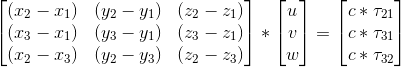求出u⃗=(u,v,w)\vec{u}=(u,v,w) 后，由正余弦关系就有了角度值了
θ=acos(1w)\theta=acos(\frac{1}{w})
α=acos(usin(acos(1w)))\alpha=acos(\frac{u}{sin(acos(\frac{1}{w}))})
当麦克风数量N&gt;3N&gt;3时，其实所有组合信息对于角度值的计算是有冗余的，这个时候可以求出所有组合的角度值，然后利用最小二乘求出最优解，这样可以利用到所有的麦克风的信息来提高角度估计的稳定性
References:
J. Benesty, J. Chen, and Y. Huang, Microphone Array Signal Processing. Berlin, Germany: Springer-Verlag, 2008.
J. Dibiase. A High-Accuracy, Low-Latency Technique for Talker Localization in Reverberent Environments using Microphone Arrays. PhD thesis, Brown University, Providence, RI, May 2000.
J.-M. Valin, F. Michaud, J. Rouat, D. Letourneau, Robust Sound Source Localization Using a Microphone Array on a Mobile Robot. Proc. IEEE/RSJ International Conference on Intelligent Robots and Systems (IROS), pp. 1228-1233, 2003.

展开全文广义互相关 GCC-PHAT
• 基于麦克风阵列声源定位系统的FPGA实现.pdf
• 在室内麦克风阵列声源定位算法的研究中,混响和噪声对定位精度影响很大,传统的声源定位算法无法在高混响和低信噪比的环境中保持较高的定位精度。为了解决这一问题,提出一种基于卷积神经网络的室内声源定位算法,该算法...
• 论述了基于麦克风阵列声源定位技术的基本原理，给出了利用FPGA实现系统各模块的设计方法。重点介绍了其原理和模块的电路实现，给出的基于FPGA设计实验结果表明，系统最大限度发挥了FPGA的优势、简化了系统设计、...FPGA
• 麦克风阵列声源定位 GCC-PHAT   版权声明：本文为博主原创文章，未经博主允许不得转载。 https://blog.csdn.net/u010592995/article/details/79735198 麦克风阵列声源定位（一） 利用麦克风阵列可以实现声源...
麦克风阵列声源定位 GCC-PHAT

版权声明：本文为博主原创文章，未经博主允许不得转载。 https://blog.csdn.net/u010592995/article/details/79735198

麦克风阵列声源定位（一）

利用麦克风阵列可以实现声源到达方向估计（direction-of-arrival (DOA) estimation），DOA估计的其中一种方法是计算到达不同阵元间的时间差，另外一种可以看这里，这篇主要介绍经典的GCC-PHAT方法

背景
简单说明问题背景，信号模型如下图，远场平面波，二元阵列要计算得到θθ，其实就是要求两个阵元接收到的信号时间差，现在问题变成到达时间差估计（Time-Difference-of-Arrival Estimation），因此，基于延时估计的DOA方法，其实也可以看做是分两步进行的，第一步是估计延时，第二步是计算角度，与之相对应的基于空间谱估计的DOA方法就是一步完成的。下面就分两步进行介绍

1.延时估计

1.1.互相关函数（cross-correlation function

计算y1(k)y1(k)与y2(k)y2(k)的时间差，可以计算两个信号的互相关函数,找到使互相关函数最大的值即是这两个信号的时间差
离散信号的互相关函数

R(τ)=E[x1(m)x2(m+τ)]R(τ)=E[x1(m)x2(m+τ)]

求时间差就是找到互相关函数最大时的点

D=argmaxR(n)D=argmaxR(n)

说的那么简单，那就用代码验证下

%%
c = 340.0;
Fs = 44100;
%%

d = 0.25;
N = 2;
mic = phased.OmnidirectionalMicrophoneElement;
% array = phased.URA([N,N],[0.0724,0.0418],'Element',mic);
array = phased.ULA(N,d,'Element',mic);

%%
% Simulate the incoming signal using the |WidebandCollector| System
% object(TM).
arrivalAng = 42;
collector = phased.WidebandCollector('Sensor',array,'PropagationSpeed',c,...
'SampleRate',Fs,'ModulatedInput',false);
signal = collector(y,arrivalAng);

x1 = signal(:,1);
x2 = signal(:,2);

N =length(x2);
xc = xcorr(x1,x2,'biased');
[k,ind] = max(xc);
an = acos((ind-N)/Fs*340/d)*180/pi

xc12 = zeros(2*N-1,1);
m = 0;
for i = -(N-1):N-1
m = m+1;
for t = 1:N
if 0<(i+t)&&(i+t)<=N
xc12(m) = xc12(m) + x2(t)*x1(t+i);
end
end
end
xc12 = xc12/N;

以上程序中的循环就是上面的定义公式，运行程序可以看到循环部分计算的互相关与直接调用matlab的xcorr结果相同（注意matlab中互相关默认没做归一化）,找到互相关函数的最大值就可以得到时间差1.2.广义互相关（generalized cross-correlation）

理论上使用上面个介绍的CCF方法就可以得到时间差，但是实际的信号会有噪声，低信噪比会导致互相关函数的峰值不够明显，这会在找极值的时候造成误差。
为了得到具有更陡峭极值的互相关函数，一般在频域使用一个加权函数来白化输入信号，这就是经典的广义互相关方法。
由维纳-辛钦定理可知，随机信号的自相关函数和功率谱密度函数服从一对傅里叶变换的关系，即x1、x2x1、x2的互功率谱可由下式计算

P(ω)=∫+∞−∞R(τ)e−jωτdτP(ω)=∫−∞+∞R(τ)e−jωτdτ

R(τ)=∫+∞−∞P(ω)ejωτdωR(τ)=∫−∞+∞P(ω)ejωτdω

这一步是把互相关函数变换到了频域，哦对，上面说到是想白化互相关函数，那就把上面第二式添加一个系数

R~(τ)=∫+∞−∞A(ω)P(ω)ejωτdωR~(τ)=∫−∞+∞A(ω)P(ω)ejωτdω

设计不同的频域系数A(ω)A(ω)对应着不同方法，这里只介绍 PHAT(phase transform)方法，即取系数如下：

A(ω)=1|P(ω)|A(ω)=1|P(ω)|

基本思想就是求时间差只需要相位信息，舍弃不相关的幅度信息以提高健壮性，可以看到当A(ω)=1A(ω)=1的情况下就是经典互相关
P(ω)P(ω)为复数，可以表示为|P(ω)|∗e−jωp|P(ω)|∗e−jωp,去掉幅度信息后，就只剩相位信息e−jωpe−jωp了，要得到相位信息，可以用P(ω)abs(P(ω))P(ω)abs(P(ω))计算，也可以直接用matlab中的angle函数计算，即angle(P(ω))angle(P(ω))，

具体得到更陡峭的峰值的理论解释如下,详情参见《麦克风阵列信号处理》P198几行代码验证下：

x1 = [1,2,3,7,9,8,3,7]';
x2 = [4,5,6,5,4,3,8,2]';

[tau,R,lag] = gccphat(x1,x2)

N = length(x1)+length(x2)-1;
NFFT = 32;
P = (fft(x1,NFFT).*conj(fft(x2,NFFT)));
A = 1./abs(P);
R_est1 = fftshift(ifft(A.*P));
range = NFFT/2+1-(N-1)/2:NFFT/2+1+(N-1)/2;
R_est1 = R_est1(range);

R_est2 = fftshift(ifft(exp(1i*angle(P))));
R_est2 = R_est2(range);


可以看到，三种不同写法得到的R_est1 、R_est2 与matlab自带函数gccphat计算得到的R相等。

那上面例子中的宽带语音信号，用GCC-PHAT方法得到具有陡峭峰值互相关函数，找到互相关最大时的点，结合采样频率Fs与与麦克风间距dFs与与麦克风间距d，就可以得到方向信息。频域计算互相关参考另一篇博客

2.角度计算

上面的内容计算了两个麦克风的延时，实际中假设阵列中麦克风个数为NN，则所有麦克风间两两组合共有N(N−1)/2N(N−1)/2对，记第kk个麦克风坐标为(xk,yk,zk)(xk,yk,zk),声源单位平面波传播向量u⃗ =(u,v,w)u→=(u,v,w),如果麦克风k,jk,j之间的延时为τkjτkj，则根据向量关系有下式，其中c为声速，

c∗τkj=−(xk→−xj→)∗u⃗ c∗τkj=−(xk→−xj→)∗u→

这样看起来不够直观，那就代入坐标写成标量形式如下：

c∗τkj=u∗(xk−xj)+v∗(yk−yj)+w∗(zk−zj)c∗τkj=u∗(xk−xj)+v∗(yk−yj)+w∗(zk−zj)

当有多个麦克风时，每两个麦克风就可以得到一组上式，N个麦克风就会有N∗(N−1)/2个等式N个麦克风就会有N∗(N−1)/2个等式，声源单位传播向量u⃗ =(u,v,w)u→=(u,v,w)有三个未知数，因此最少只需要三组等式，也就是三个麦克风就可以计算出声源方向，这里就先假定N=3N=3，可以得到方程组如下：

c∗τ21=u∗(x2−x1)+v∗(y2−y1)+w∗(z2−z1)c∗τ21=u∗(x2−x1)+v∗(y2−y1)+w∗(z2−z1)
c∗τ31=u∗(x3−x1)+v∗(y3−y1)+w∗(z3−z1)c∗τ31=u∗(x3−x1)+v∗(y3−y1)+w∗(z3−z1)
c∗τ23=u∗(x2−x3)+v∗(y2−y3)+w∗(z2−z3)c∗τ23=u∗(x2−x3)+v∗(y2−y3)+w∗(z2−z3)

写成矩阵形式求出u⃗ =(u,v,w)u→=(u,v,w)后，由正余弦关系就有了角度值了

θ=acos(1w)θ=acos(1w)

α=acos(usin(acos(1w)))α=acos(usin(acos(1w)))

当麦克风数量N>3N>3时，其实所有组合信息对于角度值的计算是有冗余的，这个时候可以求出所有组合的角度值，然后利用最小二乘求出最优解，这样可以利用到所有的麦克风的信息来提高角度估计的稳定性
展开全文• 一般来说，基于麦克风阵列声源定位算法划分为三类： 一是基于波束形成的方法；二是基于高分辨率谱估计的方法；三是基于声达时延差（TDOA）的方法。 语音定位跟踪系统基于可控波束成形技术，相比于基于...
其高科技：

http://www.keygotech.com/cn/solution/ssl/array/noise-source-location-based-on-mic-array

一般来说，基于麦克风阵列的声源定位算法划分为三类：

一是基于波束形成的方法；二是基于高分辨率谱估计的方法；三是基于声达时延差（TDOA）的方法。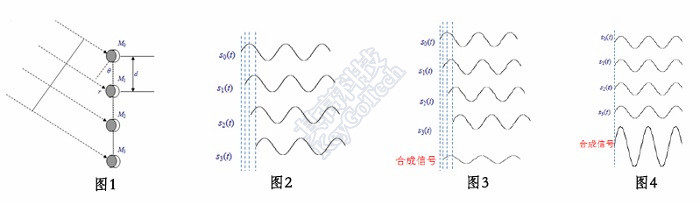语音定位跟踪系统基于可控波束成形技术，相比于基于时延的声源定位方法（TDOA），波束成形技术的抗干扰能力更强，可以在背景噪声较为复杂的环境中声源进行准确定位。并且能根据具客户的需求，对一些场景进行区分。比如识别到有人拍手，不仅可以获取拍手的位置，还能够准确识别拍手的次数，做出指定的操作。

本系统可使用硬件内部接口的信息传输方式，或通过 wifi 网络与主控程序进行通信。便捷、简单的数据传输方式，使其可以完美的与现有的智能家居或机器人产品进行整合，增加这些产品的智能度。

语音定位跟踪声源系统中，麦克风将按照进行语音定位时的最优空间位置进行排布。由于这些麦克风的空间位置不同（如图 1），故麦克风拾取到的声源的信号直接存在时间的延迟（如图 2），（注：忽略了幅值的差异）。被动语音定位跟踪系统使用的波束成形算法将各路信号进行时延后相加，获取合成信号的总能量。当信号的时延被部分抵消，获取的合成信号能量较小（如图 3），当所有信号的时延均被抵消后，获取的合成信号的总能量最大（如图 4）。获取遍历空间各个位置所对应的合成信号的总能量，当信号总能量最大时，其所对应的空间位置，即为声源的真实位置。


展开全文• ## 麦克风阵列声源定位 SRP-PHAT

万次阅读 多人点赞 2018-08-11 14:35:59
声源定位方法一般可分为两大类,一种是基于TDOA的两步算法(two-stage algorithm),一种是基于空间谱估计的一步算法，也就是这里要介绍的可控波束响应（steered-response power） steered-response power 　可控...
DOA

声源定位方法一般可分为三类,一种是基于TDOA的两步算法(two-stage algorithm)，一种是基于空间谱估计如MUSIC等，还有就是基于beamforming的方法，也就是这里要介绍的可控波束响应（steered-response power），

steered-response power

可控波束响应是利用波束形成（beamforming）的方法，对空间不同方向的声音进行增强，得到声音信号最强的方向就被认为是声源的方向。
上一篇中简单介绍了麦克风阵列的背景知识，最简单的SRP就是利用延时-累加(delay-and-sum)的方法，寻找输出能量最大的方向。
其中，语音信号为宽带信号，因此需要做宽带波束形成，这里我们在频域实现

频域宽带波束形成

频域宽带波束形成可以归类为DFT波束形成器，结构如下图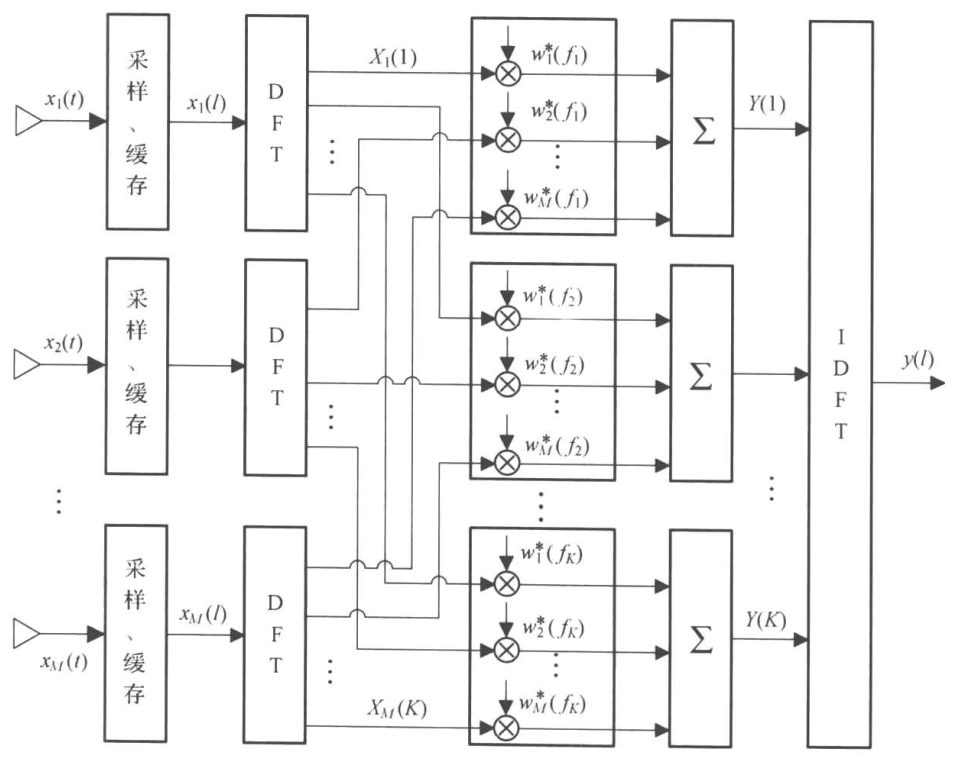频域处理也可以看做是子带处理（subband），DFT和IDFT的系数分别对应子带处理中的分析综合滤波器组，关于这一种解释，可参考《传感器阵列波束优化设计与应用》第六章。

频域宽带延时累加波束形成的基本过程就是信号分帧加窗->DFT->各频点相位补偿->IDFT
代码实现如下

nction [ DS, x1] = DelaySumURA( x,fs,N,frameLength,inc,r,angle)
%%%%%%%%%%%%%%%%%%%%%%%%%%%%%%%%%%%%%%%%%%%%%%%%%%%%%%%%%%%%%%%%%%%%
%frequency-domain delay-sum beamformer using circular array
%
%      input :
%          x : input signal ,samples * channel
%          fs: sample rate
%          N : fft length,frequency bin number
%frameLength : frame length,usually same as N
%        inc : step increment
%          r : array element radius
%      angle : incident angle
%
%     output :
%         DS : delay-sum output
%         x1 : presteered signal,same size as x
%%%%%%%%%%%%%%%%%%%%%%%%%%%%%%%%%%%%%%%%%%%%%%%%%%%%%%%%%%%%%%%%%%%%%%%%%%

c = 340;
Nele = size(x,2);
omega = zeros(frameLength,1);
H = ones(N/2+1,Nele);

theta = 90*pi/180; %固定一个俯仰角
gamma = [30 90 150 210 270 330]*pi/180;%麦克风位置
tao = r*sin(theta)*cos(angle(1)-gamma)/c;     %方位角 0 < angle <360
yds = zeros(length(x(:,1)),1);
x1 = zeros(size(x));

% frequency bin weights
% for k = 2:1:N/2+1
for k = 1:1:5000*N/fs
omega(k) = 2*pi*(k-1)*fs/N;
% steering vector
H(k,:) = exp(-1j*omega(k)*tao);
end

for i = 1:inc:length(x(:,1))-frameLength

d = fft(bsxfun(@times, x(i:i+frameLength-1,:),hamming(frameLength)));

x_fft=bsxfun(@times, d(1:N/2+1,:),H);

% phase transformed
%x_fft = bsxfun(@rdivide, x_fft,abs(d(1:N/2+1,:)));
yf = sum(x_fft,2);
Cf = [yf;conj(flipud(yf(2:N/2)))];

% 恢复延时累加的信号
yds(i:i+frameLength-1) = yds(i:i+frameLength-1)+(ifft(Cf));

% 恢复各路对齐后的信号
xf  = [x_fft;conj(flipud(x_fft(2:N/2,:)))];
x1(i:i+frameLength-1,:) = x1(i:i+frameLength-1,:)+(ifft(xf));
end
DS = yds/Nele;

end


然后遍历各个角度重复调用这个函数，测试实际录音数据，代码如下

%% SRP Estimate of Direction of Arrival at Microphone Array
% Frequency-domain delay-and-sum test
%
%%

% x = filter(Num,1,x0);
c = 340.0;

% XMOS circular microphone array radius
d = 0.0420;
% more test audio file in ../../TestAudio/ folder
path = '../../TestAudio/XMOS/room_mic5-2/';
signal = [s1,s2,s3,s4,s5,s6];
M = size(signal,2);
%%
t = 0;

% minimal searching grid
step = 1;

P = zeros(1,length(0:step:360-step));
tic
for i = 0:step:360-step
% Delay-and-sum beamforming
[ DS, x1] = DelaySumURA(signal,fs,512,512,256,d,i/180*pi);
t = t+1;
%beamformed output energy
P(t) = DS'*DS;
waitbar(i / length(step:360-step))
end
toc
close(h)
[m,index] = max(P);
figure,plot(0:step:360-step,P/max(P))
ang = (index)*step

程序中用的是圆阵，可以进行二维方向角扫描，不过这里为了简便就固定了俯仰角，只扫描方位角，结果如下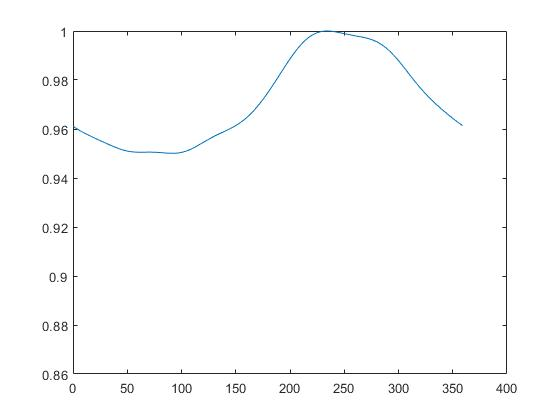结果与预期相同

PHAT加权

与GCC-PHAT方法相同，这里也可以对幅度做归一化，只保留相位信息，使得到的峰值更明显，提高在噪声及混响环境下的性能
上面代码中加上这一句

%x_fft = bsxfun(@rdivide, x_fft,abs(d(1:N/2+1,:)));

测试同样的文件，结果如下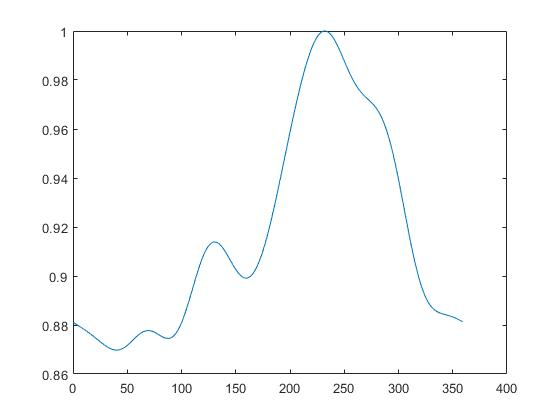对比可以看到，PHAT加权的方法性能更好

代码及测试文件在github

参考

1.《SRP-PHAT-A High-Accuracy, Low-Latency Technique for Talker Localization in Reverberant Environments Using Microphone Arrays》
2. 《传感器阵列波束优化设计与应用》

展开全文• 讲诉的是怎样利用麦克风进行声源定位。让你了解这方面的知识。对于这些有所了解。
• 麥克風陣列音源定位系統是利用麥克風陣列接收音訊，然後經由適當的演算法估算出音源入射麥克風陣列的方向角，即判斷出音源的位置方向。本專題著重於此演算法的程式撰寫，並實際測試二維及三維空間中單一音源入射的...
•研究论文
• 基于麦克风阵列声源定位聚焦算法研究，居太亮，彭启琮，基于麦克风阵列声源定位技术在通信、控制、声纳等领域已经受到广泛关注，宽带聚焦类算法是解决宽带信号定位问题的重要算法。常信息与信号处理
• 最近一直在做麦克风阵列相关处理算法的工作，今天写下来做一个总结。众所周知，麦克风的作用是将物理声学信号转化为计算机可以处理的数字信号，麦克风阵列是麦克风的集群，是有多个麦克风组成的集合。为什么要用多个...
• 针对传统方法不能准确地测量远场多声源位置的问题，提出了在近场和远场都...通过仿真实验验证了该方法在近场、远场都能准确地测量多声源位置，通过调节两个L型麦克风阵列之间的距离能得到误差在5%以下的声源定位精度。
• 麦克风阵列技术麦克风阵列技术详解声源定位延时估计角度计算波束形成波束形成模型波束形成基本理论去混响麦克风阵列结构设计声学结构确认流程 紧接上一个博客文章，此为第三部分。上一部分见：麦克风阵列技术 二 ...
• 行业分类-物理装置-基于麦克风阵列声源定位方法、装置和计算机设备.zip
• 一般的声源定位方法是基于无遮挡的线性或非线性麦克风阵列。采用头佩式麦克风阵列，考虑到背向声源麦克风的低频声波由于头盔遮挡而发生的衍射作用，针对低频波段的声音信号进行定位算法的设计和研究。该算法利用低频...
• 本文采用了嵌套线性麦克风阵列和 NI 的 DAQ 设备采集数据，用基本的 MUSIC 算法进行波达方向估计，并利用 Labview 作为软件平台，控制云台转动跟踪目标，最终完成了预期目标
• 目前基于麦克风阵列声源定位方法大致可以分为三类：基于最大输出功率的可控波束形成技术、基于高分辨率谱图估计技术和基于声音时间差（time-delay estimation，TDE）的声源定位技术。 基于TDE的算法核心在于对...算法
• 为了优化声源被动定位技术，以及降低功耗、提高精度、增强对人员不易到达的恶劣环境等区域的声源监测能力，设计了一种基于麦克风阵列声源被动定位系统。以五元十字形为麦克风阵列模型，以FPGA为核心，采用VHDL编写......